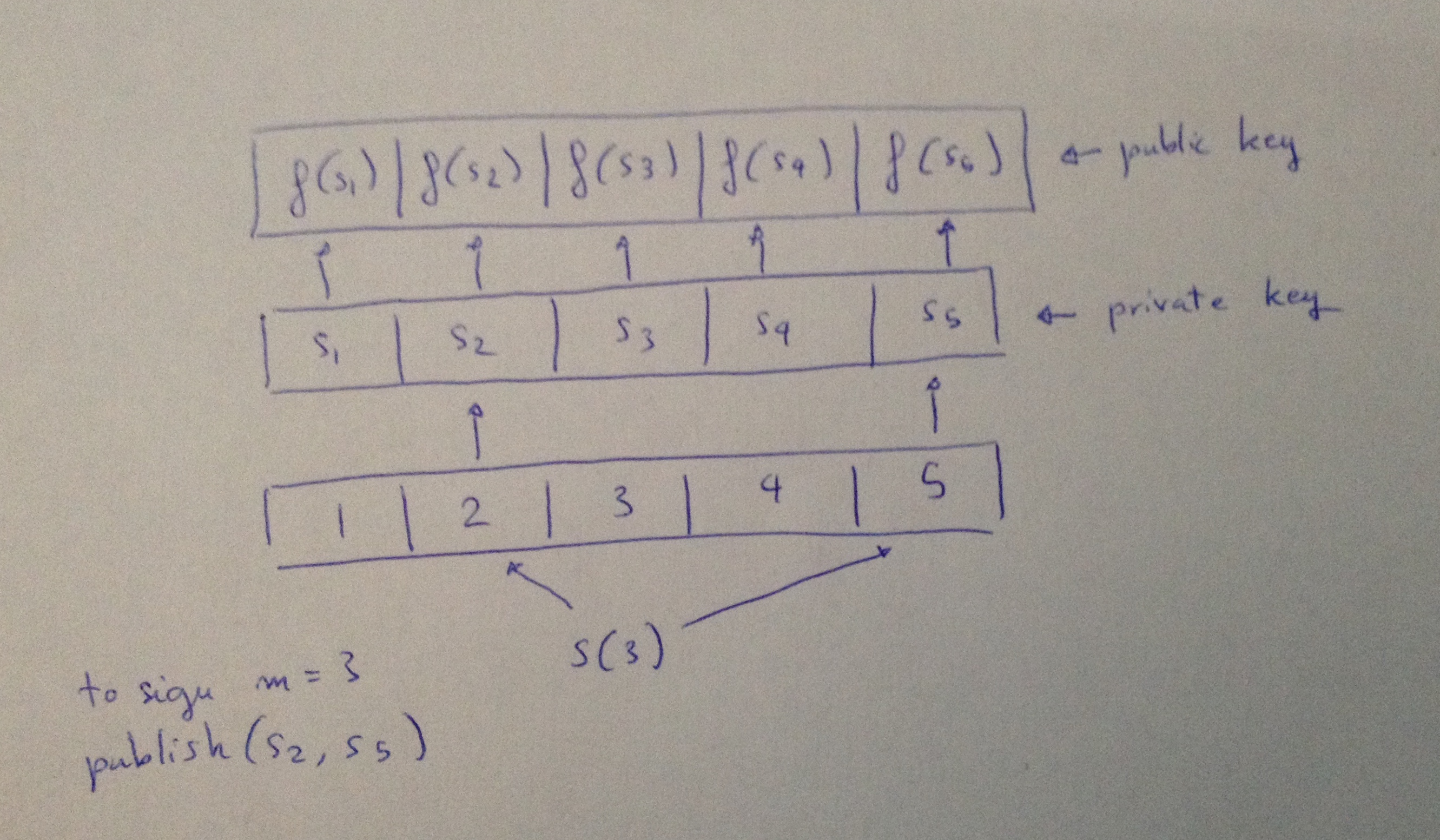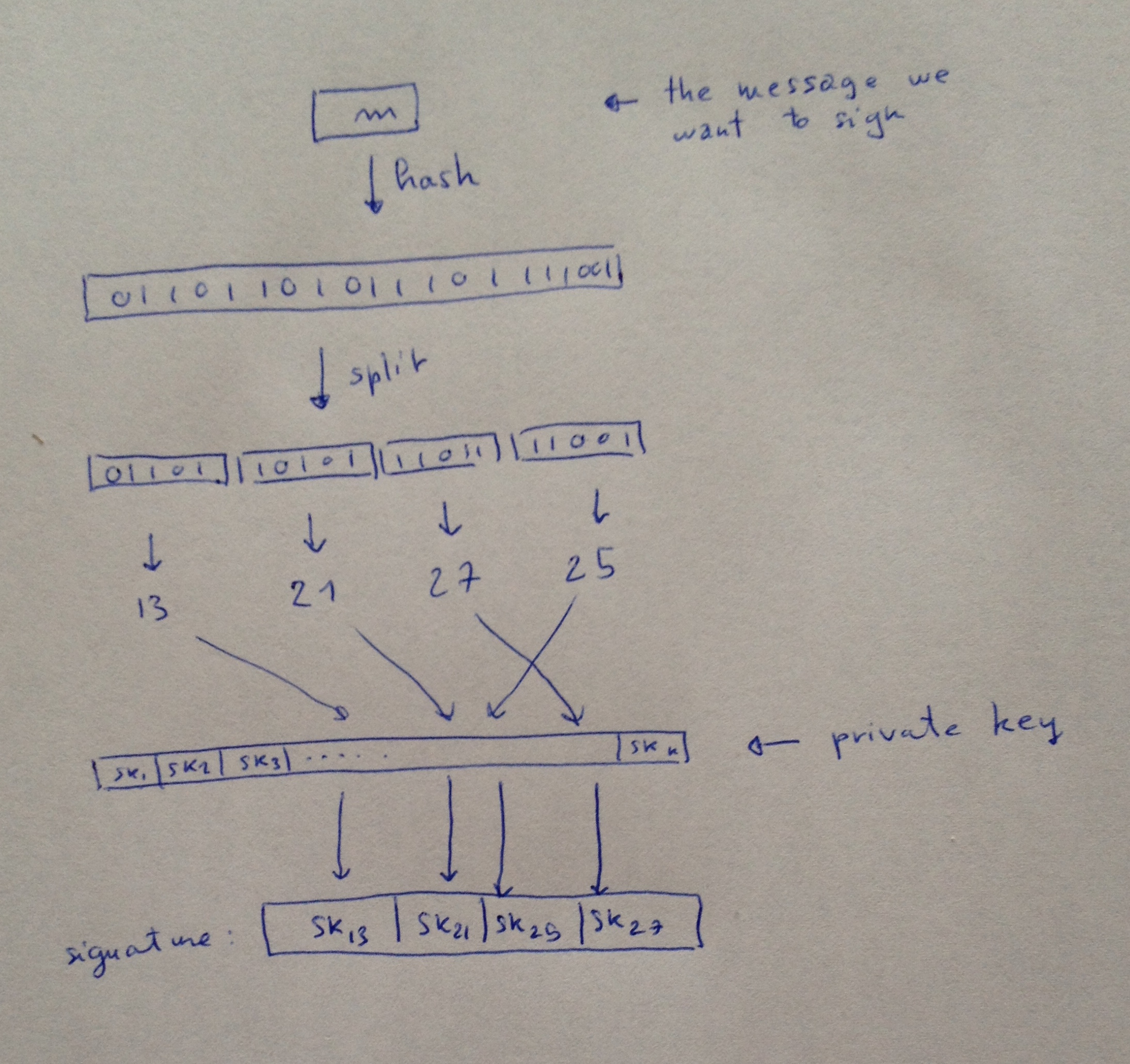If you missed the previous blogpost on OTS, go check it out first. This is about a construction a bit more useful, that allows to sign more than one signature with the same small public-key/private-key. The final goal of this series is to see how hash-based signature schemes are built. But they are not the only applications of one-time signatures (OTS) and few-times signatures (FTS).

For completeness here’s a quote of some paper about other researched applications:

One-time signatures have found applications in constructions of ordinary signature schemes [Mer87, Mer89], forward-secure signature schemes [AR00], on-line/off-line signature schemes [EGM96], and stream/multicast authentication [Roh99], among others […] BiBa broadcast authentication scheme of[Per01]

But let’s not waste time on these, today’s topic is HORS!

## HORS

HORS comes from an update of BiBa (for “Bins and Balls”), published in 2002 by the Reyzin father and son in a paper called Better than BiBa: Short One-time Signatures with Fast Signing and Verifying.

The first construction, based on one-way functions, starts very similarly to OTS: generate a list of integers that will be your private key, then hash each of these integers and you will obtain your public key.

But this time to sign, you will also need a selection function $$S$$ that will give you a list of index according to your message $$m$$. For the moment we will make abstraction of it.

In the following example, I chose the parameters $$t = 5$$ and $$k = 2$$. That means that I can sign messages $$m$$ whose decimal value (if interpreted as an integer) is smaller than $$\binom{t}{k} = 10$$. It also tells me that the length of my private key (and thus public key) will be of $$5$$ while my signatures will be of length $$2$$ (the selection function S will output 2 indexes).Using a good selection function S (a bijective function), it is impossible to sign two messages with the same elements from the private key. But still, after two signatures it should be pretty easy to forge new ones. The second construction is what we call the HORS signature scheme. It is based on “subset-resilitient” functions instead of one-way functions. The selection function $$S$$ is also replaced by another function $$H$$ that makes it infeasible to find two messages $$m_1$$ and $$m_2$$ such that $$H(m_2) \subseteq H(m_1)$$.

More than that, if we want the scheme to be a few-times signature scheme, if the signer provides $$r$$ signatures it should be infeasible to find a message $$m’$$ such that $$H(m’) \subseteq H(m_1) \cup \dots \cup H(m_r)$$. This is actually the definition of “subset-resilient”. Our selection function $$H$$ is r-subset-resilient if any attacker cannot find (even with small probability), and in polynomial time, a set of $$r+1$$ messages that would confirm the previous formula. From the paper this is the exact definition (but it basically mean what I just said)so imagine the same previous scheme:But here the selection function is not a bijection anymore, so it’s hard to reverse. So knowing the signatures of a previous set of message, it’s hard to know what messages would use such indexes.

This is done in theory by using a random oracle, in practice by using a hash function. This is why our scheme is called HORS for Hash to Obtain Random Subset.

If you’re really curious, here’s our new selection function:

to sign a message $$m$$:

1. $$h = Hash(m)$$

2. Split $$h$$ into $$h_1, \dots, h_k$$

3. Interpret each $$h_j$$ as an integer $$i_j$$

4. The signature is $$sk_{i_1}, \dots, sk_{i_k}$$

And since people seem to like my drawings: